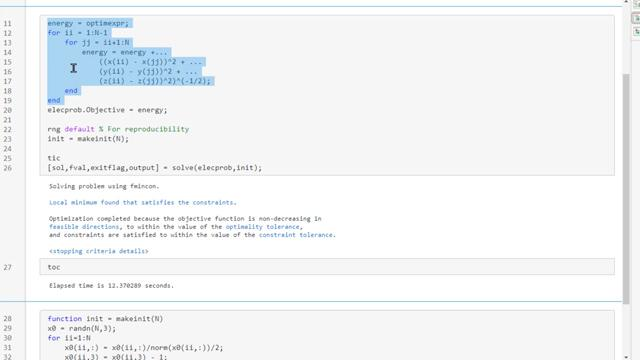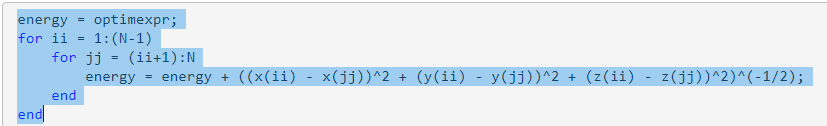# Create `for` Loop for Static Analysis

Consider the problem of placing 20 electrons in a conducting body (see Constrained Electrostatic Nonlinear Optimization Using Optimization Variables). The electrons arrange themselves in a way that minimizes their total potential energy, subject to the constraint of lying inside the body. This example focuses on the potential energy, which is naturally expressed as a nested `for` loop, and how to convert the `for` loop to perform static analysis.

### Problem Creation Without Modifications for Static Analysis

For `N` = 20 electrons, create the problem and optimization variables.

```N = 20; x = optimvar("x",N,LowerBound=-1,UpperBound=1); y = optimvar("y",N,LowerBound=-1,UpperBound=1); z = optimvar("z",N,LowerBound=-2,UpperBound=0); elecprob = optimproblem;```

The conducting body has the following constraints.

```elecprob.Constraints.spherec = (x.^2 + y.^2 + (z+1).^2) <= 1; elecprob.Constraints.plane1 = z <= -x-y; elecprob.Constraints.plane2 = z <= -x+y; elecprob.Constraints.plane3 = z <= x-y; elecprob.Constraints.plane4 = z <= x+y;```

The objective function is the potential energy of the system, which is a sum over each electron pair of the inverse of their distances:

`$energy=\sum _{i`

Define the objective function as an optimization expression.

```energy = optimexpr; for ii = 1:(N-1) for jj = (ii+1):N energy = energy + ((x(ii) - x(jj))^2 + (y(ii) - y(jj))^2 + (z(ii) - z(jj))^2)^(-1/2); end end elecprob.Objective = energy;```

Start the optimization with the electrons distributed randomly on a sphere of radius 1/2 centered at [0,0,–1].

```rng default % For reproducibility x0 = randn(N,3); for ii=1:N x0(ii,:) = x0(ii,:)/norm(x0(ii,:))/2; x0(ii,3) = x0(ii,3) - 1; end init.x = x0(:,1); init.y = x0(:,2); init.z = x0(:,3);```

Solve the problem by calling `solve`. Time the solution.

```tic [sol,fval,exitflag,output] = solve(elecprob,init)```
```Solving problem using fmincon. Local minimum found that satisfies the constraints. Optimization completed because the objective function is non-decreasing in feasible directions, to within the value of the optimality tolerance, and constraints are satisfied to within the value of the constraint tolerance. <stopping criteria details> ```
```sol = struct with fields: x: [20×1 double] y: [20×1 double] z: [20×1 double] ```
```fval = 163.0099 ```
```exitflag = OptimalSolution ```
```output = struct with fields: iterations: 94 funcCount: 150 constrviolation: 0 stepsize: 2.8395e-05 algorithm: 'interior-point' firstorderopt: 8.1308e-06 cgiterations: 0 message: 'Local minimum found that satisfies the constraints.↵↵Optimization completed because the objective function is non-decreasing in ↵feasible directions, to within the value of the optimality tolerance,↵and constraints are satisfied to within the value of the constraint tolerance.↵↵<stopping criteria details>↵↵Optimization completed: The relative first-order optimality measure, 8.090881e-07,↵is less than options.OptimalityTolerance = 1.000000e-06, and the relative maximum constraint↵violation, 0.000000e+00, is less than options.ConstraintTolerance = 1.000000e-06.' bestfeasible: [1×1 struct] objectivederivative: "reverse-AD" constraintderivative: "closed-form" solver: 'fmincon' ```
`toc`
```Elapsed time is 12.185916 seconds. ```

The solution time is over 10 seconds (your results might vary).

### Modify Problem for Efficient Static Analysis

For a visual walkthrough of these steps, watch the video.To make the optimization expression work more efficiently, extract the `for` loop and convert it to a local function. First, select the lines of code from `energy = optimexpr;` to the final `end`. Then, right-click the selected lines of code and choose Convert to Local Function.Edit the resulting function (which appears at the end of this example) as follows:

• Change the function name to `myenergy`.

• Edit the first line of code to read:

```energy = function myenergy(N, x, y, z); ```
• In the second line of code, change the initial value from `optimexpr` to 0.

To take advantage of static analysis, place the energy into the problem as the objective function by calling `fcn2optimexpr`.

```energy = fcn2optimexpr(@myenergy,N,x,y,z); elecprob.Objective = energy;```

Solve the problem by calling `solve`. Time the solution.

```tic [sol,fval,exitflag,output] = solve(elecprob,init)```
```Solving problem using fmincon. Local minimum found that satisfies the constraints. Optimization completed because the objective function is non-decreasing in feasible directions, to within the value of the optimality tolerance, and constraints are satisfied to within the value of the constraint tolerance. <stopping criteria details> ```
```sol = struct with fields: x: [20×1 double] y: [20×1 double] z: [20×1 double] ```
```fval = 163.0099 ```
```exitflag = OptimalSolution ```
```output = struct with fields: iterations: 94 funcCount: 150 constrviolation: 0 stepsize: 2.8395e-05 algorithm: 'interior-point' firstorderopt: 8.1308e-06 cgiterations: 0 message: 'Local minimum found that satisfies the constraints.↵↵Optimization completed because the objective function is non-decreasing in ↵feasible directions, to within the value of the optimality tolerance,↵and constraints are satisfied to within the value of the constraint tolerance.↵↵<stopping criteria details>↵↵Optimization completed: The relative first-order optimality measure, 8.090881e-07,↵is less than options.OptimalityTolerance = 1.000000e-06, and the relative maximum constraint↵violation, 0.000000e+00, is less than options.ConstraintTolerance = 1.000000e-06.' bestfeasible: [1×1 struct] objectivederivative: "reverse-AD" constraintderivative: "closed-form" solver: 'fmincon' ```
`toc`
```Elapsed time is 0.586535 seconds. ```

The solution time decreases considerably, to under one second.

### Compare with No Analysis

You can also solve the problem by treating the objective function as a black box. A black box objective cannot take advantage of automatic differentiation, so the solver must take finite difference steps to estimate the objective gradient. However, a black box can run quickly when the underlying function is fast to evaluate. Compare the solution time when you set `fcn2optimexpr` to create a black box objective.

```energy = fcn2optimexpr(@myenergy,N,x,y,z,Analysis="off"); elecprob.Objective = energy; tic [sol,fval] = solve(elecprob,init)```
```Solving problem using fmincon. Feasible point with lower objective function value found. Solver stopped prematurely. fmincon stopped because it exceeded the function evaluation limit, options.MaxFunctionEvaluations = 3.000000e+03. ```
```sol = struct with fields: x: [20×1 double] y: [20×1 double] z: [20×1 double] ```
```fval = 163.3910 ```
`toc`
```Elapsed time is 0.362745 seconds. ```

The solver does not complete the optimization because it exceeds the function evaluation limit. Also, the resulting `fval` is higher than in the previous two solutions. Increase the function evaluation limit and try again.

```opts = optimoptions("fmincon",MaxFunctionEvaluations=1e4); tic [sol,fval,exitflag,output] = solve(elecprob,init,Options=opts)```
```Solving problem using fmincon. Feasible point with lower objective function value found. Local minimum found that satisfies the constraints. Optimization completed because the objective function is non-decreasing in feasible directions, to within the value of the optimality tolerance, and constraints are satisfied to within the value of the constraint tolerance. <stopping criteria details> ```
```sol = struct with fields: x: [20×1 double] y: [20×1 double] z: [20×1 double] ```
```fval = 163.0099 ```
```exitflag = OptimalSolution ```
```output = struct with fields: iterations: 91 funcCount: 5666 constrviolation: 0 stepsize: 2.0317e-06 algorithm: 'interior-point' firstorderopt: 9.3405e-06 cgiterations: 0 message: 'Local minimum found that satisfies the constraints.↵↵Optimization completed because the objective function is non-decreasing in ↵feasible directions, to within the value of the optimality tolerance,↵and constraints are satisfied to within the value of the constraint tolerance.↵↵<stopping criteria details>↵↵Optimization completed: The relative first-order optimality measure, 9.294707e-07,↵is less than options.OptimalityTolerance = 1.000000e-06, and the relative maximum constraint↵violation, 0.000000e+00, is less than options.ConstraintTolerance = 1.000000e-06.' bestfeasible: [1×1 struct] objectivederivative: "finite-differences" constraintderivative: "closed-form" solver: 'fmincon' ```
`toc`
```Elapsed time is 0.610420 seconds. ```

The solution time is approximately the same as with static analysis, as is the objective function value. The `output` structure shows that the solver takes finite difference steps to estimate the objective gradient. The solver takes thousands of function evaluations, instead of fewer than 200 for the previous cases. If your function is time consuming to evaluate, this method might be inefficient.

### Conclusions

Sometimes, you can save solution time by converting a `for` loop for static analysis. Converting a `for` loop to a function is fairly simple: highlight the loop, right-click to display the context menu, select the option for creating a local function or function file, and make minor edits to the new function.

In any case, use `fcn2optimexpr` to place your `for` loop expressions into your problem. This practice allows you to obtain any future improvements in static analysis automatically, without rewriting code.

### Helper Function

The following code creates the `myenergy` helper function.

```function energy = myenergy(N, x, y, z) energy = 0; for ii = 1:(N-1) for jj = (ii+1):N energy = energy + ((x(ii) - x(jj))^2 + (y(ii) - y(jj))^2 + (z(ii) - z(jj))^2)^(-1/2); end end end```